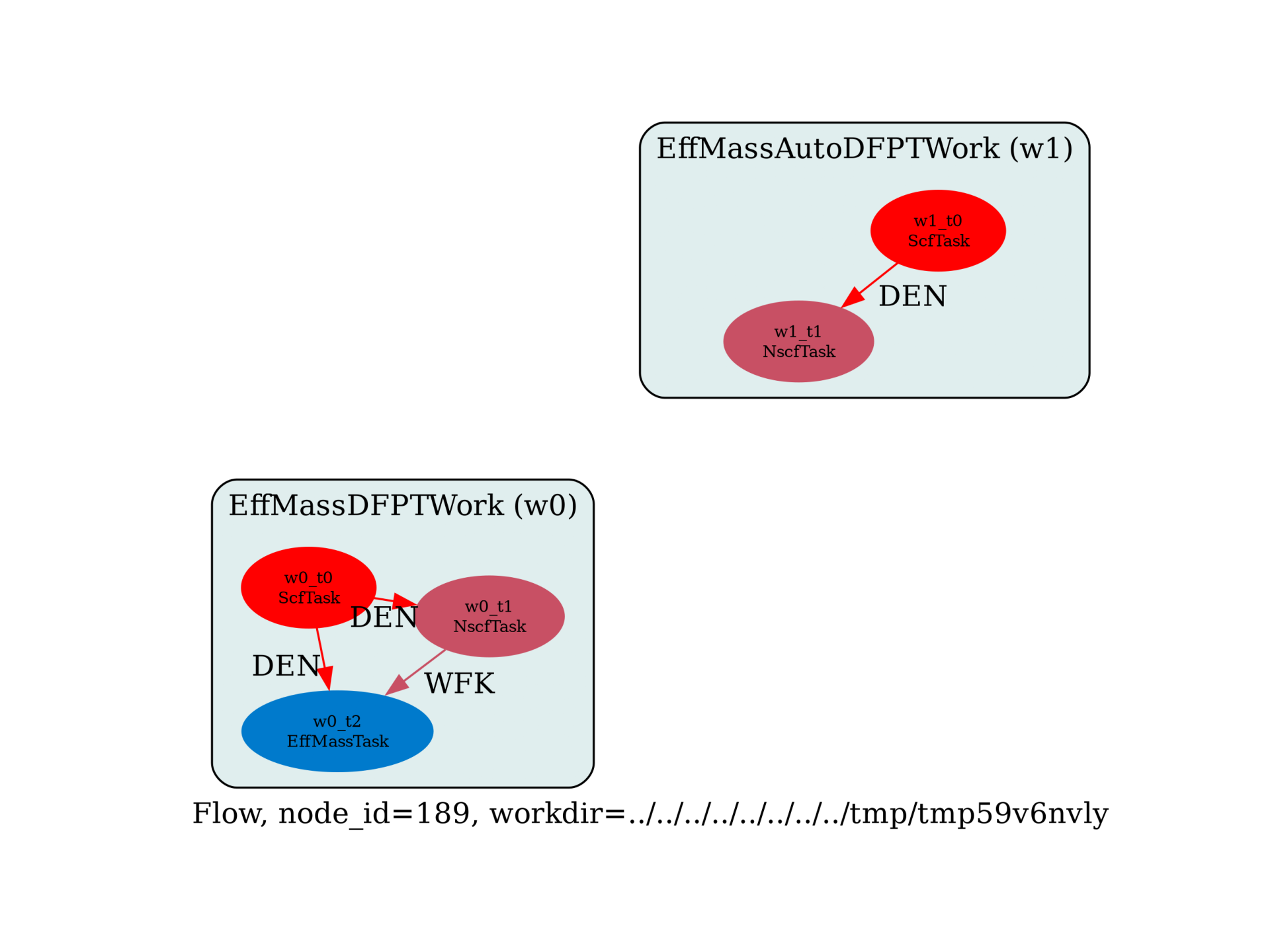# Effective masses with DFPT¶

Flow to compute effective masses with DFPT. Two options are available:

• EffMassDFPTWork –> Run DFPT calculation directly assuming the location of the band edges is already known.

• EffMassAutoDFPTWork –> Run NSCF calculation to find band edges, then use DFPT.

import sys
import os
import abipy.data as abidata
import abipy.abilab as abilab
import abipy.flowtk as flowtk

def make_scf_input(usepaw=0, nspinor=1):
"""Returns input for GS-SCF calculation."""
if nspinor == 1:
pseudos = abidata.pseudos("14si.pspnc") if usepaw == 0 else abidata.pseudos("Si.GGA_PBE-JTH-paw.xml")
else:
pseudos = abidata.pseudos("Si_r.psp8") if usepaw == 0 else abidata.pseudos("Si.GGA_PBE-JTH-paw.xml")

# https://docs.abinit.org/tests/v7/Input/t82.in
structure = dict(
ntypat=1,
natom=2,
typat=[1, 1],
znucl=14,
#acell=3 * [10.26310667319252],
acell=3 * [10.2073557], # 5.4015 Ang
rprim=[[0.0,  0.5,  0.5],
[0.5,  0.0,  0.5],
[0.5,  0.5,  0.0]],
xred=[[0.0 , 0.0 , 0.0],
[0.25, 0.25, 0.25]],
)

scf_input = abilab.AbinitInput(structure=structure, pseudos=pseudos)

# Global variables
nband = 8 if nspinor == 1 else 16
scf_input.set_vars(
ecut=8,
nband=nband,
nspinor=nspinor,
nstep=100,
tolvrs=1e-8,
)

if scf_input.ispaw:
scf_input.set_vars(pawecutdg=2 * scf_input["ecut"])

# Set k-mesh
scf_input.set_kmesh(ngkpt=[8, 8, 8], shiftk=[0, 0, 0])

return scf_input

def build_flow(options):
# Set working directory (default is the name of the script with '.py' removed and "run_" replaced by "flow_")
if not options.workdir:
options.workdir = os.path.basename(sys.argv).replace(".py", "").replace("run_", "flow_")

# Get the SCF input (without SOC)
scf_input = make_scf_input(nspinor=1, usepaw=1)

# Build the flow.
from abipy.flowtk.effmass_works import EffMassDFPTWork, EffMassAutoDFPTWork
flow = flowtk.Flow(workdir=options.workdir, manager=options.manager)

# Compute effective masses for each k in k0_list.
# effmass_bands_f90 defines the band range for each k in k0_list
# Here we are interested in the effective masses at the Gamma point for the valence bands
effmass_bands_f90 = [1, 4] if scf_input["nspinor"] == 1 else [1, 8]
work = EffMassDFPTWork.from_scf_input(scf_input, k0_list=(0, 0, 0), effmass_bands_f90=effmass_bands_f90)
flow.register_work(work)

# or use this Work to detect band edges automatically but increase ndivsm and decrease tolwfr!
work = EffMassAutoDFPTWork.from_scf_input(scf_input, ndivsm=5, tolwfr=1e-12)
flow.register_work(work)

return flow

# This block generates the thumbnails in the Abipy gallery.
# You can safely REMOVE this part if you are using this script for production runs.
__name__ = None
import tempfile
options = flowtk.build_flow_main_parser().parse_args(["-w", tempfile.mkdtemp()])
build_flow(options).graphviz_imshow()

@flowtk.flow_main
def main(options):
"""
This is our main function that will be invoked by the script.
flow_main is a decorator implementing the command line interface.
Command line args are stored in options.
"""
return build_flow(options)

if __name__ == "__main__":
sys.exit(main())Out:

/Users/gmatteo/git_repos/pymatgen/pymatgen/util/plotting.py:550: UserWarning: Matplotlib is currently using agg, which is a non-GUI backend, so cannot show the figure.
plt.show()


Run the script with:

run_effmass_dfpt -s

Total running time of the script: ( 0 minutes 0.770 seconds)

Gallery generated by Sphinx-Gallery